085-1263404

### My Journal

All things Mathematical
16 Jun 2019

### Leaving Cert Applied Maths Higher Level 1980 Solution

1. (i)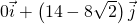,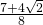hours (ii) 1.45 hours
2.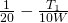3.  (ii)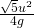4.  (ii)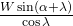(iii)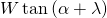(iv)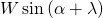5.  (b)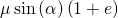6.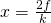7.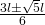8.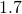m,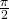s,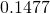s
9. (a)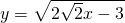(b)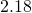m s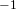10. (a)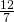(b)### Leaving Cert Applied Maths Higher Level 1981 Solution

1. (i)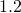m s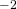and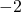m s2. “Show that” question
3. “Show that” question
4. “Show that” question
5.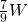,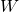and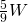6.  (a)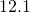percent shorter (b)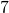rad s7.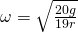8. (b) 66
9. (b)(ii)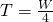and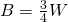(ii)10. (a)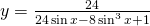(b)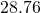m

### Leaving Cert Applied Maths Higher Level 1982 Solution

1. (i)  (a)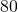m (ii)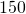s (b)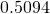N
2. (i)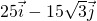(ii)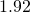km
3.  (ii)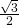(iii)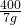m
4.  (a)and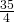m s,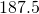kg m s(b)5.  “Show that” question
6.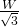for both,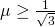7.  (ii)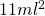8.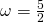rad sand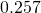s
9.  (a) (i)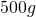Pa (ii)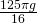N (iii) 7:1 (b)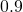10.  (a)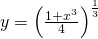(b)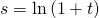and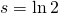m

###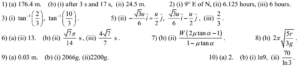This site uses Akismet to reduce spam. Learn how your comment data is processed.